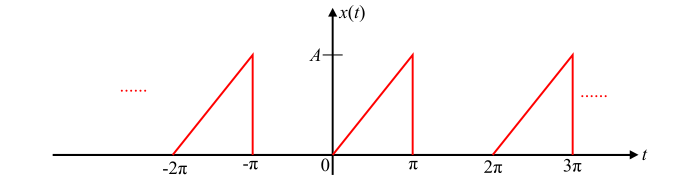# Trigonometric Fourier Series – Definition and Explanation

A periodic signal can be represented over a certain interval of time in terms of the linear combination of orthogonal functions, if these orthogonal functions are trigonometric functions, then the Fourier series representation is known as trigonometric Fourier series.

## Explanation

Consider a sinusoidal signal $x(t)=A\:sin\:\omega_{0}t$ which is periodic with time period $T$ such that $T=2\pi/ \omega_{0}$. If the frequencies of two sinusoids are integral multiples of a fundamental frequency $(\omega_{0})$, then the sum of these two sinusoids is also periodic.

We can prove that a signal $x(t)$ that is a sum of sine and cosine functions whose frequencies are integral multiples of the fundamental frequency $(\omega_{0})$, is a periodic signal.

Let the signal $x(t)$ is given by,

$$\mathrm{x(t)=a_{0}+a_{1}\:cos\:\omega_{0}t+a_{2}\:cos\:2\omega_{0}t+a_{3}\:cos\:3\omega_{0}t+....+a_{k}\:cos\:k\omega_{0}t}$$

$$\mathrm{\:\:\:\:\:\:\:\:\:\:+b_{1}\:sin\:\omega_{0}t+b_{2}\:sin\:2\omega_{0}t+b_{3}\:sin\:3\omega_{0}t+...+b_{k}\:sin\:k\omega_{0}t}$$

$$\mathrm{\Rightarrow\:x(t)=a_{0}+\sum_{n=1}^{k}a_{n}\:cos\:n\omega_{0}t+b_{n}\:sin\:n\omega_{0}t… (1)}$$

Where, $a_{0},a_{1},a_{2}....a_{k}$ and $b_{0},b_{1},b_{2}....b_{k}$ are the constants and $\omega_{0}$ is the fundamental frequency.

Again, if a signal $x(t)$ is a periodic signal, then it must satisfy the following condition −

$$\mathrm{x(t)=x(t+T);\:\:for\:all\:t}$$

Therefore,

$$\mathrm{\Rightarrow\:x(t+T)=a_{0}+\sum_{n=1}^{k}a_{n}\:cos\:n\omega_{0}(t+T)+b_{n}\:sin\:n\omega_{0}(t+T)}$$

$$\mathrm{\because\:Time\:period,T=\left ( \frac{2\pi}{\omega_{0}}\right )}$$

$$\mathrm{\Rightarrow\:x(t+T)=a_{0}+\sum_{n=1}^{k}a_{n}\:cos\:n\omega_{0}(t+\frac{2\pi}{\omega_{0}})+b_{n}\:sin\:n\omega_{0}(t+\frac{2\pi}{\omega_{0}})}$$

$$\mathrm{\Rightarrow\:x(t+T)=a_{0}+\sum_{n=1}^{k}a_{n}\:cos(n\omega_{0} t+2n\pi)+b_{n}\:sin(n\omega_{0} t+2n\pi)}$$

$$\mathrm{\because\:cos(2n\pi+\theta )=cos\:\theta \:\:and\:\:sin(2n\pi+\theta )=sin\:\theta}$$

Using these trigonometric identities, we get,

$$\mathrm{\Rightarrow\:x(t+T)=a_{0}+\sum_{n=1}^{k}a_{n}\:cos(n\omega_{0} t)+b_{n}\:sin(n\omega_{0} t)=x(t)… (2)}$$

From equation (2) it is clear that the signal $x(t)$ which is a sum of sine and cosine functions of frequencies 0,$\omega_{0},2\omega_{0},...k\omega_{0}$ is a periodic signal with a time period T. If in the expression of $x(t),k\rightarrow \infty$ then we can obtain the Fourier series representation of any periodic signal $x(t)$.

Therefore, any periodic signal can be represented as an infinite sum of sine and cosine functions which themselves are periodic signals of angular frequencies 0,$\omega_{0},2\omega_{0},...k\omega_{0}$ . This set of harmonically related sine and cosine functions form a complete set of orthogonal functions over the time interval $t$ to $(t+T)$

Hence, the trigonometric form of Fourier series can be defined as under −

The infinite series of sine and cosine terms of frequencies 0,$\omega_{0},2\omega_{0},...k\omega_{0}$ is called the trigonometric form of Fourier series and can be represented as,

$$\mathrm{x(t)=a_{0}+\sum_{n=1}^{\infty}a_{n}\:cos\: n\omega_{0} t+b_{n}\:sin\:n\omega_{0} t… (3)}$$

Where, $a_{0},a_{n}$ and $b_{n}$ are called trigonometric Fourier series confidents.

$$\mathrm{a_{0}=\frac{1}{T} \int_{t_{0}}^{(t_{0}+T)}x(t)\:dt… (4)}$$

$$\mathrm{a_{n}=\frac{2}{T} \int_{t_{0}}^{(t_{0}+T)}x(t)\:cos\:n\omega_{0}t\:dt… (5)}$$

$$\mathrm{b_{n}=\frac{2}{T} \int_{t_{0}}^{(t_{0}+T)}x(t)\:sin\:n\omega_{0}t\:dt… (6)}$$

Here,

• The coefficient $a_{0}$ is known as the DC component.

• $(a_{1}\:cos\:\omega_{0}t+b_{1}\:sin\:\omega_{0}t)$ is called the first harmonic term.

• $(a_{2}\:cos\:\omega_{0}t+b_{2}\:sin\:2\omega_{0}t)$is called the second harmonic term.

• Similarly, $(a_{n}\:cos\:n\omega_{0}t+b_{n}\:sin\:n\omega_{0}t)$ is called the nth harmonic term.

## Numerical Example

Find the trigonometric Fourier series for the waveform shown below.Solution

As we can see the given waveform is periodic with a time period $T= 2\pi$.

Mathematically, the given waveform can be described as,

$$\mathrm{x(t)=\begin{cases}(\frac{A}{\pi})t & for\:0 ≤ t ≤\:\pi\0 & for\:\pi≤ t ≤2\pi\end{cases}}$$

Let,

$$\mathrm{t_{0}=0\:\:and\:\:(t_{0}+T)= 2\pi}$$

Then, the fundamental frequency of the given function is,

$$\mathrm{\omega_{0}=\frac{2\pi}{T}=\frac{2\pi}{2\pi}=1}$$

Thus, the coefficient $a_{0}$ is given by,

$$\mathrm{a_{0}=\frac{1}{T}\int_{t_{0}}^{(t_{0}+T)}x(t)dt}$$

$$\mathrm{\Rightarrow\:a_{0}=\frac{1}{2\pi}\int_{0}^{2\pi}x(t)\:dt=\frac{1}{2\pi}\int_{0}^{\pi}(\frac{A}{\pi})t\:dt+\frac{1}{2\pi}\int_{0}^{2\pi}0\:dt=\frac{A}{2\pi^{2}}\left [ \frac{t^{2}}{2}\right ]_{0}^{\pi}=\frac{A}{4}}$$

The coefficient $a_{n}$ is given by,

$$\mathrm{a_{n}=\frac{2}{T} \int_{t_{0}}^{(t_{0}+T)}x(t)cos\:n\omega_{0}t\:\:dt}$$

$$\mathrm{\Rightarrow\:a_{n}=\frac{2}{2\pi} \int_{0}^{\pi}(\frac{A}{\pi})t\:cos\:nt\:dt=\frac{A}{\pi^{2}}\int_{0}^{\pi}t\:cos\:nt\:dt}$$

By solving the above integration, we get,

$$\mathrm{\Rightarrow\:a_{n}=\frac{A}{\pi^{2}n^{2}}[cos\:n\pi]}$$

$$\mathrm{\therefore\:a_{n}=\begin{cases}-(\frac{2A}{\pi^{2}n^{2}}) & for\:odd\:n\0 & for\:even \:n\end{cases}}$$

Similarly, the coefficient $b_{n}$ is given by,

$$\mathrm{b_{n}=\frac{2}{T}\int_{t_{0}}^{(t_{0}+T)}x(t)sin\:n\omega_{0}t\:dt}$$

$$\mathrm{\Rightarrow\:b_{n}=\frac{2}{2\pi}\int_{0}^{\pi}(\frac{A}{\pi})t\:sin\:nt\:dt=\frac{A}{\pi^{2}}\int_{0}^{\pi}t\:sin\:nt\:dt}$$

On solving this integration, we have,

$$\mathrm{b_{n}=\frac{A}{\pi^{2}}\left [-\frac{\pi\:cos\:n\pi}{n} +\left (\frac{sin\:nt}{n^{2}} \right )_{0}^{\pi} \right ]}$$

$$\mathrm{\Rightarrow\:b_{n}=-\frac{A}{n\pi}cos\:n\pi=\frac{A}{n\pi}(-1)^{n+1}}$$

$$\mathrm{\therefore\:b_{n}=\begin{cases}(\frac{A}{n\pi}) & for\:odd\:n\(-\frac{A}{n\pi}) & for\:even\:n\end{cases}}$$

Therefore, the trigonometric Fourier series is,

$$\mathrm{x(t)=a_{0}+\sum_{n=1}^{\infty}a_{n}\:cos\:n\:\omega_{0}t+b_{n}\:sin\:n\omega_{0}t}$$

$$\mathrm{\Rightarrow\:x(t)=\frac{A}{4}-\frac{2A}{\pi^{2}}\sum_{n=odd}^{\infty}\frac{cos\:nt}{n^{2}}+\frac{A}{\pi}\sum_{n=1}^{\infty}(-1)^{n+1}\cdot \frac{sin\:nt}{n}}$$